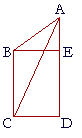# Book I.  Propositions 39 - 41

Problems

1.   a)  State the hypothesis of Proposition 39.

Equal triangles are on the same base and on the same side of it.

2.  b)  State the conclusion.

Those triangles are in the same parallels.

2.  c)  Practice Proposition 39.

2.   Proposition 40 is the partial converse of Proposition 38.  State its
2.   enunciation.

Equal triangles that are on equal bases and on the same side are in the same parallels.

3.   a)  State the hypothesis of Proposition 41.

A parallelogram and a triangle are on same base and in the same parallels.

2.  b)  State the conclusion.

The parallelogram is double the triangle.

3.  c)  Practice Proposition 41.

4.   BE is equal and parallel to CD.  EA is in a straight line with DE.
9.   Prove that quadrilateral EBCD is double triangle ABC.Since BE, CD are equal and parallel,
BC, ED are also equal and parallel;   (I. 33)
therefore the quadrilateral EBCD is a parallelogram. (Def. 14)
And it is on the same base BC, and in the same parallels
BC, AD as triangle ABC;
therefore quadrilateral EBCD is double triangle ABC.   (I. 41)

Next proposition

Copyright © 2021 Lawrence Spector

Questions or comments?

E-mail:  themathpage@yandex.com